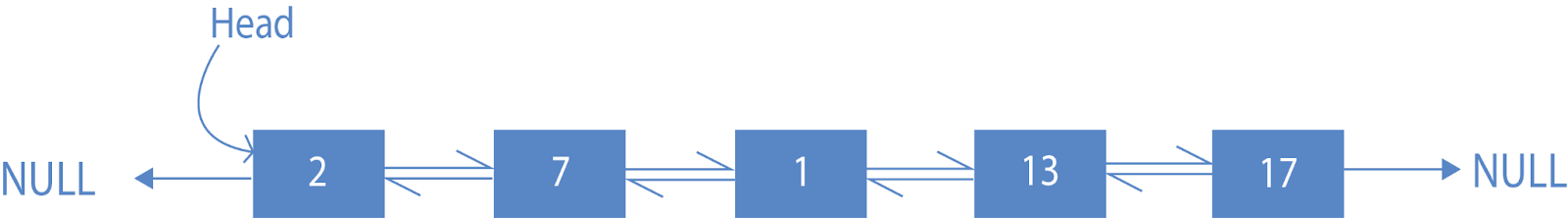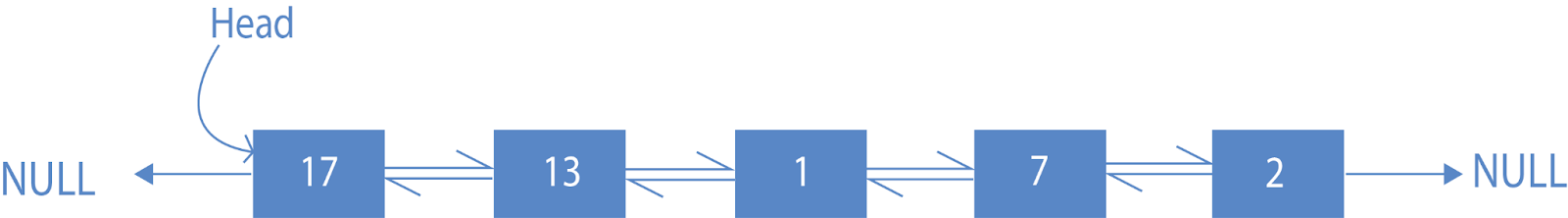# Reverse the  Doubly Linked List

## Problem Statement

Given a doubly linked list , we have reversed the given linked list.

Input:-

1<-> 2<-> 3<-> 4<->5

Output:-

5<->4<->3<->2<->1

## Explanation of the Problem Statement

We are given a doubly linked list ,where we have to reverse the linked list. As we see the input part 1-2-3-4-5 then we have to return output in reverse order of 5-4-3-2-1.

If we traverse the linked list then we see the order is  1 2 3 4 5 for the given doubly linked list, if we traverse back we get 5 4 3 2 1 , which is directly matched with the expected output.

## Approach for the solving

We are using the simple approach for reversing the doubly linked list; we are just swapping the next and prev address of nodes of its own; after that, all task gets completed, then we previous of the last node with head to access the element in the reverse side then after our task is done for the reversing the Linked List.

## Dry-Run

Let's take an example to dry-run the above implementation of the code.Here we see the Doubly Linked List 2-7-1-13-17 are given. We have given the task to reverse it to bring it below the Doubly Linked List as 17-13-1-7-2The steps are as follow for solving the problem:-

1. Create a doubly Linked List.
2. Create a reverselist function where we perform some steps:
3. First, declare the two nodes temp and curr and initialized temp as null and curr as the head.
4. Second, run a while loop to traverse the linked list and exchange the next and prev value of nodes with itself.
5. At last, after complete traversal, at reaching the end of the node, we change the head of the linked list, make the last node prev address with the head of the linked list.
6. In the end, we print the linked list by calling the print function.

Now gets with the dry-run part with the example as shown above:

1. First, we have assigned the head =2; then, we call the reverse list function. We must declare one node temp for storing the prev node value and another curr node for traversing the doubly linked list.
2. Second , now we have temp=null and curr=head , we enter the while now curr!=null ,i.e. Curr =2 now so temp=curr.prev=null , curr.prev=curr.next=7, curr.next=temp=null , curr=curr.next=7 again we perform step 2 .
3. Step-2 performs continuously till we reach the last node and the whole next and prev of the node get swapped.

4. Now at last, we change the head of the Doubly Linked List.

## Complexity

Time Complexity for the above code snippet is O(n), which we have achieved by traversing the linked list of each node and swapping the next and prev at the same time, and at the end of the list, we change the last node to head of the doubly linked list. Hence we traverse the doubly linked list as the number of nodes in it or the node’s size. So Time Complexity is O(n), where n is the size of the doubly linked list.

Space Complexity for the above code is O(1). Because we are doing changes over the given list only, we have taken an extra variable temp and current to store the node information for the particular time, which counts in the constant time. So the Space Complexity of code is O(1).

## FAQs

1. What is Time and Space Complexity for the Reverse doubly linked list?
Time Complexity is O(N), and Space Complexity is O(1).
2. What is Time Complexity?
The amount of time an algorithm takes to run as a function of the length of the input. It measures the time taken to execute each statement of code in an algorithm.
3. What is Space Complexity?
Space complexity is the amount of memory used by the algorithm to execute it entirely and produce the result.

## Key Takeaways

In this blog, we discuss the concept of swapping the next and prev of the same node. In such a manner, we get a reverse doubly linked list from the given doubly linked list. We perform the Task in the Time Complexity of O(N) and space complexity of O(1). We also discuss the real meaning of Time Complexity and Space Complexity.

Happy Coding!